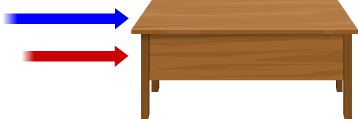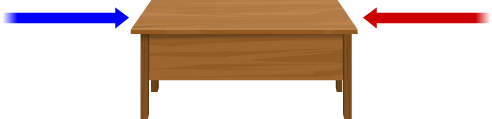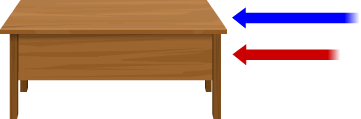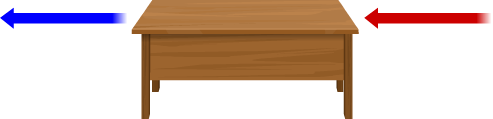Concept of Net Force

# Materials Required

• A heavy table

# The Procedure

## Real Lab Procedure

• Find a heavy object from your surroundings.
• Push the heavy object alone in any direction, say to the right as shown in the figure (The blue arrow represents the strength of your effort). Observe if the object moves.• Ask your friend to help you in pushing the object to the right as in the figure (The red arrow in the figure represents the strength of your friend). Do you feel any change while moving the object?• Ask your friend now to push the object from the opposite side to the left. Has it become easier or more difficult to move the object to the right or left?• Now both of you push the object to the left. Has it become easier to move the object?• Now one of you pull it and the other push it in the same direction.## Simulator Procedure

• Select the direction of Force 1.
• Select the Type of Force 1.
• Click on the Start button.
• If the student chooses the direction left and type push, then the table moves from left to right and increases the length of arrow for indicating it is a push.
• If the student chooses the direction left and type pull, then the table moves from right to left and decreases the length of arrow for indicating it is a pull.
• If the student chooses the direction right and type push, then the table moves from right to left and increases the length of arrow for indicating it is a push.
• If the student chooses the direction right and type pull, then the table moves from left to right and decreases the length of arrow for indicating it is a pull.
• Click on the Next button
• Select the direction of Force 1 and Force 2.
• Select the type of Force 1 and Force 2.
• Click on the Start button.
• If the student chooses Force 1: left, push and Force 2: left, push then the table moves from left to right.
• Shows inference “When forces are applied in the same direction, the total or net force is said to be equal to the sum of these forces.”
• If the student chooses Force 1: right, push and Force2: right, push then the table moves from right to left.
• Shows inference “When forces are applied in the same direction, the total or net force is said to be equal to the sum of these forces.”
• If the student chooses Force 1: left, pull and Force 2: right, push then the table moves from right to left.
• Shows inference “When forces are applied in the same direction, the total or net force is said to be equal to the sum of these forces.”
• If the student chooses Force 1: left, push and Force 2: right, pull then  the table moves from left to right.
• Shows inference “When forces are applied in the same direction, the total or net force is said to be equal to the sum of these forces.”
• If the student chooses Force 1: left, push and Force 2: right, push then screen shows a note "F1>F2" and the table moves towards F1.
• Shows inference “When forces are applied in the opposite directions, the net force is the difference of the two forces and acts in the direction of the larger force.”
• Then the screen  shows a note “F1 = F2”, then table has no movement.
• Shows inference “When forces applied in opposite directions have equal magnitudes, the net force is zero.”
• If the student chooses Force 1: left, pull and Force 2: right, pull then screen shows a note "F1
• Shows inference “When forces are applied in the opposite directions, the net force is the difference of the two forces and acts in the direction of the larger force.”
• Then the screen  shows a note “F1 = F2”, then table has no movement.
• Shows inference “When forces applied in opposite directions have equal magnitudes, the net force is zero.”
• Similarly, select all other options from the dropdown.
• Click on the Reset button to redo the experiment.

# Observations

• When you apply force alone it might be difficult to move the object.
• The object can be easily moved when both of you apply the force in the same direction either by pulling or pushing.
• It becomes difficult to move the object when both of you apply forces in opposite directions. In fact, it is possible to prevent the object from moving at all by applying equal forces in opposite directions.

# Conclusion

• When forces are applied in the same direction, the total or net force is said to be equal to the sum of these forces.
• When forces are applied in opposite directions, the net force is the difference of the two forces and acts in the direction of the larger force.
• When forces applied in opposite directions have equal magnitudes, the net force is zero.